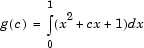## 匿名函数

### 什么是匿名函数？

`sqr = @(x) x.^2;`

`a = sqr(5)`
```a = 25```

`q = integral(sqr,0,1);`

`q = integral(@(x) x.^2,0,1);`

### 表达式中的变量

```a = 1.3; b = .2; c = 30; parabola = @(x) a*x.^2 + b*x + c;```

```clear a b c x = 1; y = parabola(x)```
```y = 31.5000```

```a = -3.9; b = 52; c = 0; parabola = @(x) a*x.^2 + b*x + c; x = 1; y = parabola(x)```
```y = 48.1000```

`save myfile.mat parabola`

### 多个匿名函数`c` 值求解以下方程，方法是合并使用两个匿名函数：

`g = @(c) (integral(@(x) (x.^2 + c*x + 1),0,1));`

1. 将被积函数编写为匿名函数，

`@(x) (x.^2 + c*x + 1)`
2. 通过将函数句柄传递到 `integral` 在从 0 到 1 的范围内计算函数，

`integral(@(x) (x.^2 + c*x + 1),0,1)`
3. 通过为整个方程构造匿名函数以提供 `c` 的值，

`g = @(c) (integral(@(x) (x.^2 + c*x + 1),0,1));`

`g(2)`
```ans = 2.3333```

### 不带输入的函数

```t = @() datestr(now); d = t()```
```d = 26-Jan-2012 15:11:47```

`d = t`
```d = @() datestr(now)```

### 带有多个输入或输出的函数

```myfunction = @(x,y) (x^2 + y^2 + x*y); x = 1; y = 10; z = myfunction(x,y)```
```z = 111 ```

```c = 10; mygrid = @(x,y) ndgrid((-x:x/c:x),(-y:y/c:y)); [x,y] = mygrid(pi,2*pi);```

```z = sin(x) + cos(y); mesh(x,y,z)```### 匿名函数的数组

```f = {@(x)x.^2; @(y)y+10; @(x,y)x.^2+y+10};```

```f = {@(x) (x.^2); @(y) (y + 10); @(x,y) (x.^2 + y + 10)};```

```x = 1; y = 10; f{1}(x) f{2}(y) f{3}(x,y)```
```ans = 1 ans = 20 ans = 21```